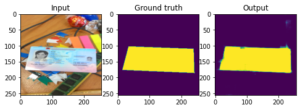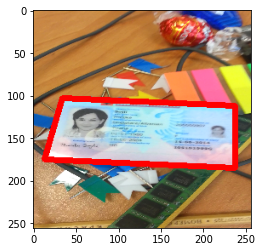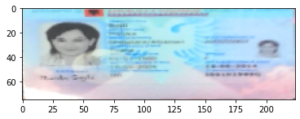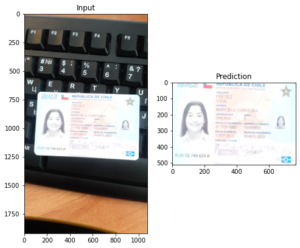November 13, 2020

# Machine Learning Solution for Extracting Information from Photographs

## Introduction

Oftentimes before we get to develop the Machine Learning Solution for Extracting Information, we need to test several approaches to see if it really is possible to solve the task and if yes, what the optimal solution would be. We develop the so-called PoC, Proofs of Concept, where we test various things applying just the minimum amount of effort to see if they work. In addition to helping us determine whether the tested tools are good enough to be developed further, we often come across interesting insights in the process.
This time we needed to create a simple PoC for a specific purpose: extracting an arbitrary document from an image. Since the task was way too complex to even create something simple, we decided to split the problem and start with a smaller task: recognizing an ID card on an image and then extracting the needed data. Luckily, there is a dataset which serves extract that purpose

## OpenCV Approach for Extracting Information

Since this task involves working with images, as usual, we tried a simple approach first: using the OpenCV library. In fact, whenever you deal with image processing, the OpenCV library cannot be avoided. If a task is not that complex, OpenCV provides a set of very useful and powerful tools to accomplish a needed goal. Thus, assuming the objects we needed to extract were mostly white rectangles, we tested a few simple ideas.

First, we tried to analyze the images applying different brightness filters, edge detection, and other tools

Using the data obtained from those filters, we tried to find contours that might satisfy some of the threshold constraints.

There were a few images where this relatively simple approach worked well (like on the image in the bottom right corner). But for most of the images we had to conclude that their colors, lightning, shadows, blurr and other conditions are so versatile, that one simple image processing algorithm can’t possibly cover it all.

Although we believe that there might exist an algorithm that can perform well under these conditions, for a PoC that must only last for only 2 days, we saw no point in continuing to work on this approach. So we turned to a little more complex approach: neural networks.

## Semantic Segmentation Approach

Specifically, we decided to try semantic segmentation for Extracting Information. That’s mostly because we have created so many of them, that creating a new one took only a few hours to write generators and train the model. We didn’t even tune the hyperparameters, since we achieved the needed purpose at the very first try.

Giving you our full code and explaining it would turn into an article worthy of Towards Data Science (this turned out to be a good tutorial for beginners. You can check the details here), but our point here is to show you that an idea can be verified relatively fast using even a complex model if you have enough expertise. Here is the gist:

1. The input to the model is an ordinary 3-channel 256×256 image.
2. This image is passed to a neural network, which also outputs a 256×256 image, but only 1-channel – an image where each pixel carries a probability of belonging to the ID card area. Here is an example of the input, true output, and model prediction:3. Then, after applying simple thresholding, we search for the contours with OpenCV: we select the one with the biggest area among those that have exactly 4 edges. Of course, most of the predicted shapes are not rectangular, so we need to smooth the edges beforehand:4. When it’s done, we reoriented the extracted rectangles so that the edges were pointed in the right direction and applied perspective transformations to level the selected area into a quadrangle:Now we were able to use this model to extract ID cards from arbitrary images by correspondingly resizing them and the predicted areas:It was a surprise even for us, but at the first try out model could achieve over 70% accuracy (for the given IoU threshold of 0.8):

We can now use this model to extract data. For instance, here is an example of what the OCR tool returns if we apply it to the result given above:

Unfortunately, the result in this case isn’t that good: although the name was extracted correctly, the expiration date was not. So, most likely, a better custom model is needed to achieve this task with an acceptable accuracy.

## Summary of Extracting Information Solution

The method we described above is not a complete solution. In fact, there are a great variety of things needed to push this to a working solution. Here are some approaches that need to be tested:

1. Using object detection instead of semantic segmentation
2. Using classifiers to filter images that don’t contain ID cards
3. Using NER for data extraction
4. Trying custom models to extract data

and many more.

Obviously, our purpose was not to demonstrate a solution. Rather it was to show that an idea sometimes can be checked very fast. Especially if you have expertise in the needed field.